# US8310388B2 - Subrange analog-to-digital converter and method thereof - Google Patents

## Info

Publication number
US8310388B2
US8310388B2 US13/050,449 US201113050449A US8310388B2 US 8310388 B2 US8310388 B2 US 8310388B2 US 201113050449 A US201113050449 A US 201113050449A US 8310388 B2 US8310388 B2 US 8310388B2
Authority
US
United States
Prior art keywords
bits
positive
capacitors
remaining
Prior art date
Legal status (The legal status is an assumption and is not a legal conclusion. Google has not performed a legal analysis and makes no representation as to the accuracy of the status listed.)
Active, expires
Application number
US13/050,449
Other versions
US20120154193A1 (en
Inventor
Soon-Jyh Chang
Ying-Zu Lin
Chun-Cheng Liu
Current Assignee (The listed assignees may be inaccurate. Google has not performed a legal analysis and makes no representation or warranty as to the accuracy of the list.)
National Cheng Kung University
Original Assignee
National Cheng Kung University
Priority date (The priority date is an assumption and is not a legal conclusion. Google has not performed a legal analysis and makes no representation as to the accuracy of the date listed.)
Filing date
Publication date
Priority to TW99144332A priority Critical
Priority to TW99144332 priority
Priority to TW099144332A priority patent/TWI452846B/en
Application filed by National Cheng Kung University filed Critical National Cheng Kung University
Assigned to NATIONAL CHENG KUNG UNIVERSITY reassignment NATIONAL CHENG KUNG UNIVERSITY ASSIGNMENT OF ASSIGNORS INTEREST (SEE DOCUMENT FOR DETAILS). Assignors: CHANG, SOON-JHY, LIN, YING-ZU, LIU, Chun-cheng
Assigned to NATIONAL CHENG KUNG UNIVERSITY reassignment NATIONAL CHENG KUNG UNIVERSITY CORRECTIVE ASSIGNMENT TO CORRECT THE ASSIGNEE'S NAME PREVIOUSLY RECORDED ON REEL 025975, FRAME 0925 Assignors: CHANG, SOON-JYH, LIN, YING-ZU, LIU, Chun-cheng
Publication of US20120154193A1 publication Critical patent/US20120154193A1/en
Publication of US8310388B2 publication Critical patent/US8310388B2/en
Application granted granted Critical
Application status is Active legal-status Critical

• 239000003990 capacitor Substances 0 claims description 145
• 230000000875 corresponding Effects 0 claims description 17
• 238000005070 sampling Methods 0 claims description 10
• 238000006243 chemical reaction Methods 0 description 5
• 238000000034 methods Methods 0 description 2

## Images

••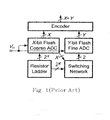•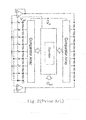•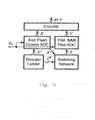•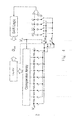•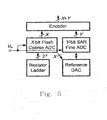•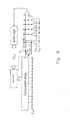•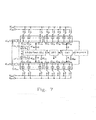••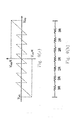•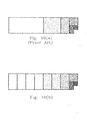•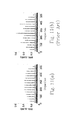•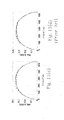## Classifications

• HELECTRICITY
• H03BASIC ELECTRONIC CIRCUITRY
• H03MCODING; DECODING; CODE CONVERSION IN GENERAL
• H03M1/00Analogue/digital conversion; Digital/analogue conversion
• H03M1/12Analogue/digital converters
• H03M1/14Conversion in steps with each step involving the same or a different conversion means and delivering more than one bit
• H03M1/145Conversion in steps with each step involving the same or a different conversion means and delivering more than one bit the steps being performed sequentially in series-connected stages
• HELECTRICITY
• H03BASIC ELECTRONIC CIRCUITRY
• H03MCODING; DECODING; CODE CONVERSION IN GENERAL
• H03M1/00Analogue/digital conversion; Digital/analogue conversion
• H03M1/12Analogue/digital converters
• H03M1/34Analogue value compared with reference values
• H03M1/36Analogue value compared with reference values simultaneously only, i.e. parallel type
• H03M1/361Analogue value compared with reference values simultaneously only, i.e. parallel type having a separate comparator and reference value for each quantisation level, i.e. full flash converter type
• H03M1/362Analogue value compared with reference values simultaneously only, i.e. parallel type having a separate comparator and reference value for each quantisation level, i.e. full flash converter type the reference values being generated by a resistive voltage divider
• H03M1/365Analogue value compared with reference values simultaneously only, i.e. parallel type having a separate comparator and reference value for each quantisation level, i.e. full flash converter type the reference values being generated by a resistive voltage divider the voltage divider being a single resistor string
• HELECTRICITY
• H03BASIC ELECTRONIC CIRCUITRY
• H03MCODING; DECODING; CODE CONVERSION IN GENERAL
• H03M1/00Analogue/digital conversion; Digital/analogue conversion
• H03M1/12Analogue/digital converters
• H03M1/34Analogue value compared with reference values
• H03M1/38Analogue value compared with reference values sequentially only, e.g. successive approximation type
• H03M1/46Analogue value compared with reference values sequentially only, e.g. successive approximation type with digital/analogue converter for supplying reference values to converter
• H03M1/466Analogue value compared with reference values sequentially only, e.g. successive approximation type with digital/analogue converter for supplying reference values to converter using switched capacitors
• HELECTRICITY
• H03BASIC ELECTRONIC CIRCUITRY
• H03MCODING; DECODING; CODE CONVERSION IN GENERAL
• H03M1/00Analogue/digital conversion; Digital/analogue conversion
• H03M1/12Analogue/digital converters
• H03M1/34Analogue value compared with reference values
• H03M1/38Analogue value compared with reference values sequentially only, e.g. successive approximation type
• H03M1/46Analogue value compared with reference values sequentially only, e.g. successive approximation type with digital/analogue converter for supplying reference values to converter
• H03M1/466Analogue value compared with reference values sequentially only, e.g. successive approximation type with digital/analogue converter for supplying reference values to converter using switched capacitors
• H03M1/468Analogue value compared with reference values sequentially only, e.g. successive approximation type with digital/analogue converter for supplying reference values to converter using switched capacitors in which the input S/H circuit is merged with the feedback DAC array

## Abstract

The configurations and adjusting method of a subrange analog-to-digital converter (ADC) are provided. The provided subrange ADC includes a X.5-bit flash ADC, a Y-bit SAR ADC and a (X+Y)-bit segmented capacitive digital-to-analog converter (DAC).

## Description

FIELD OF THE INVENTION

The application claims the benefit of Taiwan Patent Application No. 099144332, filed on Dec. 16, 2010, in the Taiwan Intellectual Property Office, the disclosures of which are incorporated herein in their entirety by reference.

The present invention relates to a subrange analog-to-digital converter (ADC). More particularly, it relates to a subrange ADC including a X.5-bit flash ADC, a Y-bit successive approximation analog-to-digital converter (SAR ADC) and a (X+Y)-bit segmented capacitive digital-to-analog converter (DAC).

BACKGROUND OF THE INVENTION

Generally speaking, the binary weighted capacitor array has a better linearity than the split capacitor array or the C-2C capacitor array so as to avoid the complex digital correction. But a drawback of the binary weighted capacitor array is that the capacitance value of its maximum bit is a sum of all the remaining bits. That means, to add a bit, the total capacitance value of the whole capacitor array will become doubled. When a high resolution version is designed, an overly large input capacitance value will limit the input bandwidth and result in that the capacitor switching requires even larger energy consumption. For a SAR ADC, after a comparison is ended and before the next comparison begins, the voltage value of the DAC in the SAR ADC must be confirmed to be settled enough to reach the required accuracy. During the whole conversion procedure, the capacitor having the maximum weight in the capacitor array (being one half of the whole capacitance value in the capacitor array) requires the longest settling time, and it is the main bottleneck of the operational speed of the SAR ADC. If the flash ADC is employed to speed up the first several capacitors with the maximum weights, then the whole subrange ADC only requires going through a large voltage variation once. As for the operations thererafter, since the capacitance of the capacitor with the maximum weight in the fine SAR ADC is significantly reduced, the settling time required is relatively shortened so as to have the advantages in speed.

Therefore, it is the intention of the present invention to combine the flash ADC with the SAR ADC so as to draw on the strong points to offset the weaknesses and to have the advantages of both the flash ADC and the SAR ADC.

Keeping the drawbacks of the prior arts in mind, and employing experiments and research full-heartily and persistently, the applicant finally conceived a subrange analog-to-digital converter and a method thereof.

SUMMARY OF THE INVENTION

It is therefore an object of the present invention to provide a subrange ADC includes a X.5-bit flash ADC, a Y-bit SAR ADC and a (X+Y)-bit segmented capacitive digital-to-analog converter (DAC), the flash ADC controls thermometer coarse capacitors of the DAC and the SAR ADC controls the binary fine capacitors; compared with a pure binary DAC, the thermometer DAC improves differential non-linearity (DNL), and due to that the flash ADC engages the analog-to-digital conversions of the X bits simultaneously, the sampling speed of the subrange ADC as a whole is also raised.

According to the first aspect of the present invention, a subrange analog-to-digital converter (ADC) with M bits comprises a positive and a negative input terminals, a first capacitor array having 2(2(N+1)−2) equivalent capacitors, each of which has a first and a second terminals, divided into N groups representing the first N bits of the M bits counted from a most significant bit (MSB) to a least significant bit (LSB), a successive approximation ADC (SAR ADC) including a second capacitor array having a plurality of bits and at least one capacitor with a first and a second terminals, wherein each the bit of the first and the second capacitor arrays electrically connects with at least one of the capacitors, the first terminal electrically connects with one of the positive and negative input terminals, and the second terminal switchably connects with a first and a second reference voltage sources to selectively receive a first and a second reference voltages, and a flash ADC receiving the first and the second reference voltages and a positive and a negative input voltages from the positive and the negative input terminals, generating two output signal sets, each of which has (2(N+1)−2) output signals, and simultaneously determining whether to adjust respective potential levels of the N bits represented by the N groups according to the two output signal sets.

According to the second aspect of the present invention, a subrange analog-to-digital converter (ADC) with M bits comprises a positive and a negative input terminals, a first capacitor array having 2(2(N+1)−2) capacitors, each of which has a first and a second terminals, divided into N groups representing the first N bits of M bits counted from a most significant bit (MSB) to a least significant bit (LSB), wherein each the bit electrically connects with at least one of the capacitors, the first terminal electrically connects with one of the positive and negative input terminals, and the second terminal switchably connects with a first and a second reference voltage sources to selectively receive a first and a second reference voltages, and a flash ADC comprising a reference voltage generating circuit formed by 2(N+1)−2 resistors electrically connected in series, receiving the first and the second reference voltages and a positive and a negative input voltages from the positive and the negative input terminals, and simultaneously determining whether to adjust potential levels of the bits of the N groups and a voltage value of the at least one of the capacitors, wherein except each of the first and the last resistors has a relatively higher first resistance, each of the remaining 2(N+1)−4 resistors has a relatively lower second resistance to generate a reference voltage required by an offset tolerance capability of the reference voltage generating circuit.

According to the third aspect of the present invention, a method for adjusting a subrange analog-to-digital converter (ADC) comprises steps of: providing 2(2(N+1)−2) capacitors, each of which has a first and a second terminals, and which capacitors are divided into N groups, wherein the N groups represent N bits, each of which electrically connects with at least one of the capacitors, the first terminal receives an input signal, and the second terminal selectively receives one of a first and a second reference voltages; comparing respectively a positive and a negative voltage values generated via the input signal with two sets of divided voltages to generate two sets of output signals; and determining simultaneously whether to adjust a voltage value of each the capacitor of each the bit of the N groups according to the two sets of 2(2(N+1)−2) output signals.

The present invention may best be understood through the following descriptions with reference to the accompanying drawings, in which:

BRIEF DESCRIPTION OF THE DRAWINGS

FIG. 1 is a schematic diagram of a conventional subrange ADC;

FIG. 2 is a schematic circuit diagram of a 6-bit embodiment of the conventional subrange ADC;

FIG. 3 is a schematic diagram of a subrange ADC comprising a flash ADC and a SAR ADC;

FIG. 4 is a 6-bit embodiment of the subrange ADC as shown in FIG. 3;

FIG. 5 is a schematic diagram of a subrange ADC comprising a flash ADC and a SAR ADC according to the first preferred embodiment of the present invention;

FIG. 6 is a schematic circuit diagram of a 6-bit subrange ADC comprising a flash ADC and a SAR ADC according to the first preferred embodiment of the present invention;

FIG. 7 is a schematic circuit diagram of a 9-bit subrange ADC comprising a 3.5-bit flash ADC and a 6-bit SAR ADC according to the first preferred embodiment of the present invention;

FIGS. 8( a) and 8(b) respectively show a timing diagram of a conventional SAR ADC and a timing diagram of a subrange ADC according to the present invention as shown in FIG. 7;

FIGS. 9( a) and 9(b) respectively show a waveform diagram depicting a residual plot after going through the flash ADC and a circuit diagram of a reference voltage generating circuit according to the first preferred embodiment of the present invention;

FIGS. 10( a) and 10(b) respectively show a schematic diagram of a binary weighted capacitor array according to a conventional SAR ADC and a schematic diagram of a segmented capacitor array according to the first preferred embodiment of the present invention;

FIGS. 11( a) and 11(b) respectively show a schematic diagram of a DNL of rms of the subrange ADC according to the first preferred embodiment of the present invention and a schematic diagram of a DNL of rms of a conventional SAR ADC; and

FIGS. 11( c) and 11(d) respectively show a schematic diagram of an INL of rms of the subrange ADC according to the first preferred embodiment of the present invention and a schematic diagram of an INL of rms of a conventional SAR ADC.

DETAILED DESCRIPTION OF THE PREFERRED EMBODIMENT

FIG. 7 shows a schematic diagram of a 9-bit subrange ADC comprising a 3.5-bit flash ADC and a 6-bit SAR ADC according to the first preferred embodiment of the present invention. According to the first preferred embodiment of the present invention, a subrange analog-to-digital converter (ADC) with M bits (e.g., an M-bit ADC) is provided and comprises a positive and a negative input terminals, a first capacitor array having 2(2(N+1)−2) equivalent capacitors, each of which has a first and a second terminals, divided into N groups representing the first N bits of the M bits counted from a most significant bit (MSB) to a least significant bit (LSB), a successive approximation ADC (SAR ADC) including a second capacitor array having a plurality of bits and at least one capacitor with a first and a second terminals, wherein each the bit of the first and the second capacitor arrays electrically connects with at least one of the capacitors (Cci=Cc(i+1)=CF5, 2CFj=CF(j+1), where i=1-13 and j=1-4), the first terminal electrically connects with one of the positive input terminal (receiving Vinp) and the negative input terminal (receiving Vinn), and the second terminal switchably connects with a first and a second reference voltage sources to selectively receive a first and a second reference voltages, and a flash ADC receiving the first reference voltage (Vrefp) and the second reference voltage (Vrefn) and a positive voltage (Vinp) and a negative input voltage (Vinn) from the positive and the negative input terminals, generating two output signal sets, each of which has (2(N+1)−2) output signals, and simultaneously determining whether to adjust respective potential levels of the N bits represented by the N groups according to the two output signal sets.

As shown in FIG. 7, the subrange ADC further comprises a first and a second bootstrapped switches, an error correction circuit (DEC) and a comparator electrically connected to the positive and the negative terminals, wherein the positive and the negative input terminals receive an input signal, the input signal is a differential signal (generating the Vinp and the Vinn accordingly), the first and the second bootstrapped switches are respectively connected between the positive input terminal and the comparator, and between the negative input terminal and the comparator for completely passing the differential signal through the positive and the negative input terminals, the SAR ADC includes a first digital control circuit (not shown), the flash ADC includes a second digital control circuit (not shown), and the error correction circuit electrically connects with the SAR ADC and the flash ADC and generates a digital code matched to the input signal. The flash ADC is an N.5-bit flash ADC (here, 3.5-bit), generates N+1 output signals (here, 4 bits) and generates the digital code corresponding to the first N bits (here, 3 bits) via the error correction circuit (DEC) such that a generation of an offset is tolerated.

In FIG. 7, the second capacitor array is configured to be one of the remaining M−N bits and the remaining M−N−1 bits (here, M−N−1 bits, i.e. 9−3−1=5 bits) of the M bits, the comparator has a comparison result, the comparison result and a binary search algorithm are used to adjust respective potential levels of one of the remaining M−N bits and the remaining M−N−1 bits (here, adjusting M−N−1 bits, i.e. adjusting 9−3−1=5 bits of potential levels) of the M bits to generate the digital code, and a top plate sampling method is used to obtain the M−N bits of the digital code when the second capacitor array is configured to be the remaining M−N−1 bits.

FIGS. 8( a) and 8(b) respectively show a timing diagram of a conventional SAR ADC and a timing diagram of a subrange ADC according to the present invention as shown in FIG. 7. Comparing FIG. 8( b) with FIG. 8( a), one could make a comparison between the timing sequences of a subrange ADC proposed in the present invention and a pure SAR ADC. Since the flash ADC of the proposed subrange ADC is employed to speed up the first several capacitors with the maximum weights (e.g., it is shown as 3 here) such that the whole subrange ADC only requires going through a large voltage variation once. As for the operations thererafter, since the capacitance of the capacitor with the maximum weight in the fine SAR ADC is significantly reduced, the settling time required is relatively shortened and the following several conversions becoming stable more quickly can be speed up to obtain an efficiency in speed.

FIGS. 9( a) and 9(b) respectively shows a waveform diagram depicting a residual plot after going through the flash ADC and a circuit diagram of a reference voltage generating circuit according to the first preferred embodiment of the present invention. FIG. 9( a) is a residual plot generated after the proposed subrange ADC going through the first stage processing of the flash ADC. This plot is quite similar to the plot of a pipelined ADC, and the only difference is that the residue is not enlarged. Since this is not an integer bits structure, so that this structure could stand a certain extent of input voltage offset of the comparator. The advantage of this design of the present invention is that the accuracy of the flash ADC is only required to be maintained at 3 bits instead of to be raised to 9 bits in the present embodiment. In FIG. 9( b), a reference voltage generating circuit comprising 2(N+1)−2 resistors electrically connected in series of the flash ADC is shown, and except the first and the last resistors have a respective relatively higher first resistance (3R), the remaining 2(N+1)−4 resistors all have a respective relatively lower second resistance (2R) to generate a reference voltage (that is the input voltage value at the turning point of the residue plot), which is required so as to realize FIG. 9( a) and is required to ensure an offset tolerance capability of the reference voltage generating circuit.

FIGS. 10 (a) and 10(b) are related to the conversions of capacitor arrays. Conventionally, the binary weighted capacitor array usually will have the worst differential nonlinearity (DNL) efficiency when the maximum bit is switched, but in the subrange ADC proposed in the present invention, the pure binary weighted form has been transformed into the segmented form (large capacitors are using thermometer code and the small capacitors are binary weighted) since the thermometer code outputted by the comparator array of the flash ADC is used. This kind of design could effectively improve the efficiency of DNL. Besides, one of the advantages of using the flash ADC as the front stage in the proposed subrange ADC is that the first several large capacitors are switched at the same time. For a SAR ADC, after a comparison is ended and before the next comparison begins, the voltage value of the DAC in the SAR ADC must be confirmed to be settled enough to reach the required accuracy. Here, the subrange ADC only requires going through a large voltage variation once. As for the operations thererafter, there is an advantage in speed since the capacitance of the capacitor with the maximum weight in the fine SAR ADC is significantly reduced.

The aforementioned first preferred embodiment of the present invention can be further generalized to become the second preferred embodiment of the present invention, which is a subrange analog-to-digital converter (ADC) with M bits (e.g., an M-bit ADC) and comprises a positive and a negative input terminals, a first capacitor array having 2(2(N+1)−2) capacitors, each of which has a first and a second terminals, divided into N groups representing the first N bits of M bits counted from a most significant bit (MSB) to a least significant bit (LSB), wherein each the bit electrically connects with at least one of the capacitors (Cci=Cc(i+1)=CF5, 2CF(j)=CF(j+1), where i=1-13 and j=1-4), the first terminal electrically connects with one of the positive input terminal (receiving Vinp) and the negative input terminal (receiving Vinn), and the second terminal switchably connects with a first and a second reference voltage sources to selectively receive a first reference voltage (Vrefp) and a second reference voltage (Vrefn), and a flash ADC comprising a reference voltage generating circuit formed by 2(N+1)−2 resistors electrically connected in series, receiving the first reference voltage (Vrefp) and the second reference voltage (Vrefn) and a positive input voltage (Vinp) and a negative input voltage (Vinn) from the positive and the negative input terminals, and simultaneously determining whether to adjust potential levels of the bits of the N groups and a voltage value of the at least one of the capacitors, wherein except each of the first and the last resistors has a relatively higher first resistance (e.g., 3R, see FIG. 9( b)), each of the remaining 2(N+1)−4 resistors has a relatively lower second resistance (e.g., 2R, see FIG. 9( b)) to generate a reference voltage required by an offset tolerance capability of the reference voltage generating circuit to stand for a relatively large offset of the positive and the negative input voltages. The 2(2(N+1)−2) capacitors are equivalent capacitors, in which each the first terminal of a first set of the 2(2(N+1)−2) capacitors is electrically connected to the positive input terminal, each the first terminal of the other set of the 2(2(N+1)−2) capacitors is electrically connected to the negative input terminal and each of the first set and the other set of the 2(2(N+1)−2) capacitors has (2(N+1)−2) capacitors.

The aforementioned first and second preferred embodiments of the present invention can be further generalized to become the third preferred embodiment of the present invention, which is a method for adjusting a subrange analog-to-digital converter (ADC) and comprises steps of: providing 2(2(N+1)−2) capacitors, each of which has a first and a second terminals, and which capacitors are divided into N groups, wherein the N groups represent N bits, each of which electrically connects with at least one of the capacitors, the first terminal receives an input signal, and the second terminal selectively receives one of a first and a second reference voltages; comparing respectively a positive and a negative voltage values generated via the input signal with two sets of divided voltages to generate two sets of output signals; and determining simultaneously whether to adjust a voltage value of each the capacitor of each the bit of the N groups according to the two sets of 2(2(N+1)−2) output signals, wherein each of the two sets of divided voltages has (2(N+1)−2) voltages and each of the two sets of output signals has (2(N+1)−2) output signals.

The above-mentioned method proposed according to the third preferred embodiment of the present invention further comprises a step of generating N digital codes respectively corresponding to the N bits according to the voltage value of each the capacitor representative of each the bit of the N groups after an adjustment in the determining step is finished.

In the aforementioned method proposed according to the third preferred embodiment of the present invention, the subrange ADC has M bits counted from a most significant bit (MSB) to a least significant bit (LSB), and the above-mentioned method further comprises steps of: providing a capacitor array having at least one capacitor with a first and a second terminals and configured to be one of the remaining (M−N) bits and the remaining (M−N−1) bits of the M bits, wherein each the bit electrically connects with the at least one capacitor, the first terminal receives the input signal and the second terminal selectively receives one of a first and a second reference voltages; applying a binary search algorithm to adjust a potential level of each bit in the one of the remaining M−N bits and the remaining M−N−1 bits of the M bits to generate additional digital codes of one of (M−N) bits and (M−N−1) bits corresponding to the one of the remaining (M−N) bits and the remaining (M−N−1) bits; and applying a top plate sampling method to obtain the digital codes of M−N bits when the second capacitor array is configured to be the remaining M−N−1 bits.

In the above-mentioned method proposed according to the third preferred embodiment of the present invention, wherein the providing step further comprises a step of providing a voltage divider, wherein all of the capacitors have an equivalent capacitance and the two sets of divided voltages are generated by the voltage divider when the first and the second reference voltages are respectively applied thereacross.

FIGS. 11( a) and 11(b) respectively shows a schematic diagram of a DNL of root mean square (rms) of the subrange ADC according to the first preferred embodiment of the present invention and a schematic diagram of a DNL of rms of a conventional SAR ADC. FIGS. 11( c) and 11(d) respectively shows a schematic diagram of an INL of rms of the subrange ADC according to the first preferred embodiment of the present invention and a schematic diagram of an INL of rms of a conventional SAR ADC. FIGS. 11( a)-11(d) are the results of simulation and analysis via using the model set up by the Matlab software. A mismatch is added to the capacitor (a standard deviation is 3%). There are in total 10,000 times of simulations, the rms results of the conventional method are shown in FIGS. 11( a) and 11(c), and the rms results according to the first preferred embodiment of the present invention are shown in FIGS. 11( b) and 11(d). It could be observed from FIGS. 11( a)-11(d) that the DNL of the subrange ADC proposed according to the first preferred embodiment of the present invention is improved significantly while compared with the DNL of the conventional SAR ADC, although there seems no significant change between the INL of the proposed subrange ADC and that of the conventional SAR ADC.

EMBODIMENTS

1. A subrange analog-to-digital converter (ADC) with M bits, comprising:

a positive and a negative input terminals;

a first capacitor array having 2(2(N+1)−2) equivalent capacitors, each of which has a first and a second terminals, divided into N groups representing the first N bits of the M bits counted from a most significant bit (MSB) to a least significant bit (LSB);

a successive approximation ADC (SAR ADC) including a second capacitor array having a plurality of bits and at least one capacitor with a first and a second terminals, wherein each the bit of the first and the second capacitor arrays electrically connects with at least one of the capacitors, the first terminal electrically connects with one of the positive and negative input terminals, and the second terminal switchably connects with a first and a second reference voltage sources to selectively receive a first and a second reference voltages; and

a flash ADC receiving the first and the second reference voltages and a positive and a negative input voltages from the positive and the negative input terminals, generating two output signal sets, each of which has (2(N+1)−2) output signals, and simultaneously determining whether to adjust respective potential levels of the N bits represented by the N groups according to the two output signal sets.

2. A converter according to Embodiment 1 further comprising a first and a second bootstrapped switches, an error correction circuit and a comparator electrically connected to the positive and the negative terminals, wherein the positive and the negative input terminals receive an input signal, the input signal is a differential signal, the first and the second bootstrapped switches are respectively connected between the positive input terminal and the comparator, and between the negative input terminal and the comparator for completely passing the differential signal through the positive and the negative input terminals, the SAR ADC includes a first digital control circuit, the flash ADC includes a second digital control circuit, and the error correction circuit electrically connects with the SAR ADC and the flash ADC and generates a digital code matched to the input signal.

3. A converter according to Embodiment 1 or 2, wherein the second capacitor array is configured to be one of the remaining M−N bits and the remaining M−N−1 bits of the M bits, the comparator has a comparison result, the comparison result and a binary search algorithm are used to adjust respective potential levels of one of the remaining M−N bits and the remaining M−N−1 bits of the M bits to generate the digital code, and a top plate sampling method is used to obtain the M−N bits of the digital code when the second capacitor array is configured to be the remaining M−N−1 bits.

4. A converter according to anyone of the above-mentioned Embodiments, wherein the flash ADC comprises a reference voltage generating circuit formed by 2(N+1)−2 resistors electrically connected in series, and except the first and the last resistors have a respective relatively higher first resistance, the remaining 2(N+1)−4 resistors all have a respective relatively lower second resistance to generate a reference voltage required by an offset tolerance capability of the reference voltage generating circuit.

5. A converter according to anyone of the above-mentioned Embodiments, wherein the flash ADC is an N.5-bit flash ADC, generates N+1 output signals and generates the digital code corresponding to the first N bits via the error correction circuit such that a generation of an offset is tolerated.

6. A subrange analog-to-digital converter (ADC) with M bits, comprising:

a positive and a negative input terminals;

a first capacitor array having 2(2(N+1)−2) capacitors, each of which has a first and a second terminals, divided into N groups representing the first N bits of M bits counted from a most significant bit (MSB) to a least significant bit (LSB), wherein each the bit electrically connects with at least one of the capacitors, the first terminal electrically connects with one of the positive and negative input terminals, and the second terminal switchably connects with a first and a second reference voltage sources to selectively receive a first and a second reference voltages; and

a flash ADC comprising a reference voltage generating circuit formed by 2(N+1)−2 resistors electrically connected in series, receiving the first and the second reference voltages and a positive and a negative input voltages from the positive and the negative input terminals, and simultaneously determining whether to adjust potential levels of the bits of the N groups and a voltage value of the at least one of the capacitors, wherein except each of the first and the last resistors has a relatively higher first resistance, each of the remaining 2(N+1)−4 resistors has a relatively lower second resistance to generate a reference voltage required by an offset tolerance capability of the reference voltage generating circuit.

7. A converter according to Embodiment 6, wherein the 2(2(N+1)−2) capacitors are equivalent capacitors, in which each the first terminal of a first set of the 2(2(N+1)−2) capacitors is electrically connected to the positive input terminal, each the first terminal of the other set of the 2(2(N+1)−2) capacitors is electrically connected to the negative input terminal and each of the first set and the other set of the 2(2(N+1)−2) capacitors has (2(N+1)−2) capacitors.

8. A converter according to Embodiment 6 or 7 further comprising a successive approximation ADC (SAR ADC) including a second capacitor array having a plurality of bits and at least one of the capacitors with a first and a second terminals, wherein each the bit of the first and the second capacitor arrays electrically connects with at least one the capacitors, the first terminal electrically connects with one of the positive and negative input terminals, and the second terminal switchably connects with a first and a second reference voltage sources to selectively receive a first and a second reference voltages.

9. A converter according to anyone of the above-mentioned Embodiments further comprising a first and a second bootstrapped switches, an error correction circuit and a comparator electrically connected to the positive and the negative terminals, wherein the positive and the negative input terminals receive an input signal, the input signal is a differential signal, the first and the second bootstrapped switches are respectively connected between the positive input terminal and the comparator, and between the negative input terminal and the comparator for completely passing the differential signal through the positive and the negative input terminals, the SAR ADC includes a first digital control circuit, the flash ADC includes a second digital control circuit, and the error correction circuit electrically connects with the SAR ADC and the flash ADC and generates a digital code matched to the input signal.

10. A converter according to anyone of the above-mentioned Embodiments, wherein the second capacitor array is configured to be one of the remaining M−N bits and the remaining M−N−1 bits of the M bits, the comparator has a comparison result, the comparison result and a binary search algorithm are used to adjust respective potential levels of one of the remaining M−N bits and the remaining M−N−1 bits of the M bits to generate the digital code, and a top plate sampling method is used to obtain the M−N bits of the digital code when the second capacitor array is configured to be the remaining M−N−1 bits.

11. A converter according to anyone of the above-mentioned Embodiments, wherein the flash ADC is an N.5-bit flash ADC, generates N+1 output signals and generates the digital code corresponding to the first N bits via the error correction circuit such that a generation of an offset is tolerated.

12. A method for adjusting a subrange analog-to-digital converter (ADC), comprising steps of:

providing 2(2(N+1)−2) capacitors, each of which has a first and a second terminals, and which capacitors are divided into N groups, wherein the N groups represent N bits, each of which electrically connects with at least one of the capacitors, the first terminal receives an input signal, and the second terminal selectively receives one of a first and a second reference voltages;

comparing respectively a positive and a negative voltage values generated via the input signal with two sets of divided voltages to generate two sets of output signals; and

determining simultaneously whether to adjust a voltage value of each the capacitor of each the bit of the N groups according to the two sets of 2(2(N+1)−2) output signals.

13. A method according to Embodiment 12, wherein each of the two sets of divided voltages has (2(N+1)−2) voltages and each of the two sets of output signals has (2(N+1)−2) output signals.

14. A method according to Embodiment 12 or 13 further comprising a step of generating N digital codes respectively corresponding to the N bits according to the voltage value of each the capacitor representative of each the bit of the N groups after an adjustment in the determining step is finished.

15. A method according to anyone of the above-mentioned Embodiments, wherein the subrange ADC has M bits counted from a most significant bit (MSB) to a least significant bit (LSB), further comprising steps of:

providing a capacitor array having at least one capacitor with a first and a second terminals and configured to be one of the remaining (M−N) bits and the remaining (M−N−1) bits of the M bits, wherein each the bit electrically connects with the at least one capacitor, the first terminal receives the input signal and the second terminal selectively receives one of a first and a second reference voltages;

applying a binary search algorithm to adjust a potential level of each bit in the one of the remaining M−N bits and the remaining M−N−1 bits of the M bits to generate additional digital codes of one of (M−N) bits and (M−N−1) bits corresponding to the one of the remaining (M−N) bits and the remaining (M−N−1) bits; and

applying a top plate sampling method to obtain the digital codes of M−N bits when the second capacitor array is configured to be the remaining M−N−1 bits.

16. A method according to anyone of the above-mentioned Embodiments, wherein the providing step further comprises a step of providing a voltage divider, wherein all of the capacitors have an equivalent capacitance and the two sets of divided voltages are generated by the voltage divider when the first and the second reference voltages are respectively applied thereacross.

According to the aforementioned descriptions, the present invention provides a subrange ADC includes a X.5-bit flash ADC, a Y-bit SAR ADC and a (X+Y)-bit segmented capacitive digital-to-analog converter (DAC), the flash ADC controls thermometer coarse capacitors of the DAC and the SAR ADC controls the binary fine capacitors; compared with a pure binary DAC, the thermometer DAC improves differential non-linearity (DNL) generated while the large capacitors are switching, and due to that the flash ADC engages the analog-to-digital conversions of the X bits simultaneously, the sampling speed of the subrange ADC as a whole is also raised so as to possess the non-obviousness and the novelty.

While the invention has been described in terms of what are presently considered to be the most practical and preferred embodiments, it is to be understood that the invention need not be limited to the disclosed embodiment. On the contrary, it is intended to cover various modifications and similar arrangements included within the spirit and scope of the appended claims, which are to be accorded with the broadest interpretation so as to encompass all such modifications and similar structures. Therefore, the above description and illustration should not be taken as limiting the scope of the present invention which is defined by the appended claims.

## Claims (16)

1. A subrange analog-to-digital converter (ADC) with M bits, comprising:
a positive and a negative input terminals;
a first capacitor array having 2(2(N+1)−2) equivalent capacitors, each of which has a first and a second terminals, divided into N groups representing the first N bits of the M bits counted from a most significant bit (MSB) to a least significant bit (LSB);
a successive approximation ADC (SAR ADC) including a second capacitor array having a plurality of bits and at least one capacitor with a first and a second terminals, wherein each the bit of the first and the second capacitor arrays electrically connects with at least one of the capacitors, the first terminal electrically connects with one of the positive and negative input terminals, and the second terminal switchably connects with a first and a second reference voltage sources to selectively receive a first and a second reference voltages; and
a flash ADC receiving the first and the second reference voltages and a positive and a negative input voltages from the positive and the negative input terminals, generating two output signal sets, each of which has (2(N+1)−2) output signals, and simultaneously determining whether to adjust respective potential levels of the N bits represented by the N groups according to the two output signal sets.
2. A converter according to claim 1 further comprising a first and a second bootstrapped switches, an error correction circuit and a comparator electrically connected to the positive and the negative terminals, wherein the positive and the negative input terminals receive an input signal, the input signal is a differential signal, the first and the second bootstrapped switches are respectively connected between the positive input terminal and the comparator, and between the negative input terminal and the comparator for completely passing the differential signal through the positive and the negative input terminals, the SAR ADC includes a first digital control circuit, the flash ADC includes a second digital control circuit, and the error correction circuit electrically connects with the SAR ADC and the flash ADC and generates a digital code matched to the input signal.
3. A converter according to claim 2, wherein the second capacitor array is configured to be one of the remaining M−N bits and the remaining M−N−1 bits of the M bits, the comparator has a comparison result, the comparison result and a binary search algorithm are used to adjust respective potential levels of one of the remaining M−N bits and the remaining M−N−1 bits of the M bits to generate the digital code, and a top plate sampling method is used to obtain the M−N bits of the digital code when the second capacitor array is configured to be the remaining M−N−1 bits.
4. A converter according to claim 1, wherein the flash ADC comprises a reference voltage generating circuit formed by 2(N+1)−2 resistors electrically connected in series, and except the first and the last resistors have a respective relatively higher first resistance, the remaining 2(N+1)−4 resistors all have a respective relatively lower second resistance to generate a reference voltage required by an offset tolerance capability of the reference voltage generating circuit.
5. A converter according to claim 1, wherein the flash ADC is an N.5-bit flash ADC, generates N+1 output signals and generates the digital code corresponding to the first N bits via the error correction circuit such that a generation of an offset is tolerated.
6. A subrange analog-to-digital converter (ADC) with M bits, comprising:
a positive and a negative input terminals;
a first capacitor array having 2(2(N+1)−2) capacitors, each of which has a first and a second terminals, divided into N groups representing the first N bits of M bits counted from a most significant bit (MSB) to a least significant bit (LSB), wherein each the bit electrically connects with at least one of the capacitors, the first terminal electrically connects with one of the positive and negative input terminals, and the second terminal switchably connects with a first and a second reference voltage sources to selectively receive a first and a second reference voltages; and
a flash ADC comprising a reference voltage generating circuit formed by 2(N+1)−2 resistors electrically connected in series, receiving the first and the second reference voltages and a positive and a negative input voltages from the positive and the negative input terminals, and simultaneously determining whether to adjust potential levels of the bits of the N groups and a voltage value of the at least one of the capacitors, wherein except each of the first and the last resistors has a relatively higher first resistance, each of the remaining 2(N+1)−4 resistors has a relatively lower second resistance to generate a reference voltage required by an offset tolerance capability of the reference voltage generating circuit.
7. A converter according to claim 6, wherein the 2(2(N+1)−2) capacitors are equivalent capacitors, in which each the first terminal of a first set of the 2(2(N+1)−2) capacitors is electrically connected to the positive input terminal, each the first terminal of the other set of the 2(2(N+1)−2) capacitors is electrically connected to the negative input terminal and each of the first set and the other set of the 2(2(N+1)−2) capacitors has (2(N+1)−2) capacitors.
8. A converter according to claim 6 further comprising a successive approximation ADC (SAR ADC) including a second capacitor array having a plurality of bits and at least one of the capacitors with a first and a second terminals, wherein each the bit of the first and the second capacitor arrays electrically connects with at least one the capacitors, the first terminal electrically connects with one of the positive and negative input terminals, and the second terminal switchably connects with a first and a second reference voltage sources to selectively receive a first and a second reference voltages.
9. A converter according to claim 8 further comprising a first and a second bootstrapped switches, an error correction circuit and a comparator electrically connected to the positive and the negative terminals, wherein the positive and the negative input terminals receive an input signal, the input signal is a differential signal, the first and the second bootstrapped switches are respectively connected between the positive input terminal and the comparator, and between the negative input terminal and the comparator for completely passing the differential signal through the positive and the negative input terminals, the SAR ADC includes a first digital control circuit, the flash ADC includes a second digital control circuit, and the error correction circuit electrically connects with the SAR ADC and the flash ADC and generates a digital code matched to the input signal.
10. A converter according to claim 9, wherein the second capacitor array is configured to be one of the remaining M−N bits and the remaining M−N−1 bits of the M bits, the comparator has a comparison result, the comparison result and a binary search algorithm are used to adjust respective potential levels of one of the remaining M−N bits and the remaining M−N−1 bits of the M bits to generate the digital code, and a top plate sampling method is used to obtain the M−N bits of the digital code when the second capacitor array is configured to be the remaining M−N−1 bits.
11. A converter according to claim 9, wherein the flash ADC is an N.5-bit flash ADC, generates N+1 output signals and generates the digital code corresponding to the first N bits via the error correction circuit such that a generation of an offset is tolerated.
12. A method for adjusting a subrange analog-to-digital converter (ADC), comprising steps of:
providing 2(2(N+1)−2) capacitors, each of which has a first and a second terminals, and which capacitors are divided into N groups, wherein the N groups represent N bits, each of which electrically connects with at least one of the capacitors, the first terminal receives an input signal, and the second terminal selectively receives one of a first and a second reference voltages;
comparing respectively a positive and a negative voltage values generated via the input signal with two sets of divided voltages to generate two sets of output signals; and
determining simultaneously whether to adjust a voltage value of each the capacitor of each the bit of the N groups according to the two sets of 2(2(N+1)−2) output signals.
13. A method according to claim 12, wherein each of the two sets of divided voltages has (2(N+1)−2) voltages and each of the two sets of output signals has (2(N+1)−2) output signals.
14. A method according to claim 12 further comprising a step of generating N digital codes respectively corresponding to the N bits according to the voltage value of each the capacitor representative of each the bit of the N groups after an adjustment in the determining step is finished.
15. A method according to claim 14, wherein the subrange ADC has M bits counted from a most significant bit (MSB) to a least significant bit (LSB), further comprising steps of:
providing a capacitor array having at least one capacitor with a first and a second terminals and configured to be one of the remaining (M−N) bits and the remaining (M−N−1) bits of the M bits, wherein each the bit electrically connects with the at least one capacitor, the first terminal receives the input signal and the second terminal selectively receives one of a first and a second reference voltages;
applying a binary search algorithm to adjust a potential level of each bit in the one of the remaining M−N bits and the remaining M−N−1 bits of the M bits to generate additional digital codes of one of (M−N) bits and (M−N−1) bits corresponding to the one of the remaining (M−N) bits and the remaining (M−N−1) bits; and
applying a top plate sampling method to obtain the digital codes of M−N bits when the second capacitor array is configured to be the remaining M−N−1 bits.
16. A method according to claim 12, wherein the providing step further comprises a step of providing a voltage divider, wherein all of the capacitors have an equivalent capacitance and the two sets of divided voltages are generated by the voltage divider when the first and the second reference voltages are respectively applied thereacross.
US13/050,449 2010-12-16 2011-03-17 Subrange analog-to-digital converter and method thereof Active 2031-05-31 US8310388B2 (en)

## Priority Applications (3)

Application Number Priority Date Filing Date Title
TW99144332A 2010-12-16
TW99144332 2010-12-16
TW099144332A TWI452846B (en) 2010-12-16 2010-12-16 Segmented analog-to-digital converter and method thereof

## Publications (2)

Publication Number Publication Date
US20120154193A1 US20120154193A1 (en) 2012-06-21
US8310388B2 true US8310388B2 (en) 2012-11-13

# Family

## Family Applications (1)

Application Number Title Priority Date Filing Date
US13/050,449 Active 2031-05-31 US8310388B2 (en) 2010-12-16 2011-03-17 Subrange analog-to-digital converter and method thereof

## Country Status (2)

US (1) US8310388B2 (en)
TW (1) TWI452846B (en)

## Cited By (9)

* Cited by examiner, † Cited by third party
Publication number Priority date Publication date Assignee Title
US20120326900A1 (en) * 2011-06-24 2012-12-27 Mediatek Inc. Successive approximation register analog to digital converter and conversion method thereof
US20130027232A1 (en) * 2011-07-29 2013-01-31 Mediatek Singapore Pte. Ltd. Analog-to-digital converters and analog-to-digital conversion methods
US8395538B2 (en) * 2011-06-20 2013-03-12 Texas Instruments Incorporated High speed resistor-DAC for SAR DAC
US8400343B1 (en) * 2011-10-18 2013-03-19 Himax Technologies Limited Pipeline analog to digital converter with split-path level shifting technique
US20130182803A1 (en) * 2012-01-18 2013-07-18 Kabushiki Kaisha Toshiba Analog-digital converter and receiver
US20140035772A1 (en) * 2012-08-06 2014-02-06 Realtek Semiconductor Corp. Successive approximation analog-to-digital converter using capacitor array with sub-capacitors configured by capacitor disassembling and related method thereof
US8760336B2 (en) * 2012-07-11 2014-06-24 Electronics And Telecommunications Research Institute Mixed mode analog to digital converter and method of operating the same
US8884801B1 (en) * 2013-11-21 2014-11-11 Inphi Corporation High-speed analog-to-digital conversion system with flash assisted parallel SAR architecture
US9362939B1 (en) * 2014-12-31 2016-06-07 Texas Instruments Incorporated Reduction of input dependent capacitor DAC switching current in flash-SAR analog-to-digital converters

## Families Citing this family (12)

* Cited by examiner, † Cited by third party
Publication number Priority date Publication date Assignee Title
US9007253B2 (en) * 2011-12-21 2015-04-14 Realtek Semiconductor Corp. Successive-approximation-register analog-to-digital converter and method thereof
TWI536747B (en) * 2013-10-04 2016-06-01 瑞昱半導體股份有限公司 Analog-to-digital conversion apparatus and method thereof
US20140306689A1 (en) 2013-04-10 2014-10-16 Texas Instruments, Incorporated High resolution current pulse analog measurement
CN103905049B (en) * 2014-03-11 2017-11-03 中国科学院半导体研究所 A kind of high-speed flash adds alternately comparison expression gradually-appoximant analog-digital converter
US10291249B2 (en) * 2016-07-18 2019-05-14 Analog Devices, Inc. Common mode rejection in a reservoir capacitor SAR converter
US10135459B2 (en) 2016-10-25 2018-11-20 Analog Devices, Inc. ADC with capacitive difference circuit and digital sigma-delta feedback
US10298252B2 (en) 2016-11-13 2019-05-21 Analog Devices, Inc. Dynamic anti-alias filter for analog-to-digital converter front end
US10327659B2 (en) 2016-11-13 2019-06-25 Analog Devices, Inc. Quantization noise cancellation in a feedback loop
EP3514965A1 (en) * 2018-01-19 2019-07-24 Socionext Inc. Analogue-to-digital converter circuitry
US10516411B1 (en) 2018-07-11 2019-12-24 Analog Devices Global Unlimited Company Common mode rejection in reservoir capacitor analog-to-digital converter
US10461762B1 (en) * 2018-08-01 2019-10-29 Qualcomm Incorporated Successive approximation register analog-to-digital converter chopping
US10355709B1 (en) 2018-08-24 2019-07-16 Analog Devices, Inc. Multiplexed sigma-delta analog-to-digital converter

## Citations (13)

* Cited by examiner, † Cited by third party
Publication number Priority date Publication date Assignee Title
US5014056A (en) * 1988-05-11 1991-05-07 Analog Devices Kk A/D converter with a main range up/down counter and a subrange A/D converter
US5099240A (en) * 1990-09-17 1992-03-24 Motorola Inc. Subranging adc with error correction through increased fine step span and noise reducing layout
US5400029A (en) * 1992-09-09 1995-03-21 Seiko Instruments Inc. Analog-digital conversion circuit device and analog-digital conversion method
US5482163A (en) * 1994-12-27 1996-01-09 Hoffman; Kenneth L. Last event indicator
US5621409A (en) * 1995-02-15 1997-04-15 Analog Devices, Inc. Analog-to-digital conversion with multiple charge balance conversions
US5861831A (en) * 1996-12-23 1999-01-19 Analog Devices, Inc. Intermediate frequency (IF) sampling clock-to-clock auto-ranging analog-to-digital converter (ADC) and method
US5973632A (en) * 1998-03-03 1999-10-26 Powerchip Semiconductor Corp. Sub-range flash analog-to-digital converter
US6204790B1 (en) * 1999-06-10 2001-03-20 Nortel Networks Limited Stacked digital-to-analog converter and methods of performing digital-to-analog conversion
US6570523B1 (en) * 2002-02-13 2003-05-27 Intersil Americas Inc. Analog to digital converter using subranging and interpolation
US6831585B2 (en) * 2002-05-24 2004-12-14 Broadcom Corporation Distributed averaging analog to digital converter topology
US7091891B2 (en) * 2004-04-28 2006-08-15 Analog Devices, Inc. Calibration of analog to digital converter by means of multiplexed stages
US7710184B2 (en) * 2006-02-08 2010-05-04 Broadcom Corporation ISI reduction technique
US7724174B2 (en) * 2008-10-07 2010-05-25 Himas Media Solutions, Inc. Successive approximation ADC with binary error tolerance mechanism

## Patent Citations (13)

* Cited by examiner, † Cited by third party
Publication number Priority date Publication date Assignee Title
US5014056A (en) * 1988-05-11 1991-05-07 Analog Devices Kk A/D converter with a main range up/down counter and a subrange A/D converter
US5099240A (en) * 1990-09-17 1992-03-24 Motorola Inc. Subranging adc with error correction through increased fine step span and noise reducing layout
US5400029A (en) * 1992-09-09 1995-03-21 Seiko Instruments Inc. Analog-digital conversion circuit device and analog-digital conversion method
US5482163A (en) * 1994-12-27 1996-01-09 Hoffman; Kenneth L. Last event indicator
US5621409A (en) * 1995-02-15 1997-04-15 Analog Devices, Inc. Analog-to-digital conversion with multiple charge balance conversions
US5861831A (en) * 1996-12-23 1999-01-19 Analog Devices, Inc. Intermediate frequency (IF) sampling clock-to-clock auto-ranging analog-to-digital converter (ADC) and method
US5973632A (en) * 1998-03-03 1999-10-26 Powerchip Semiconductor Corp. Sub-range flash analog-to-digital converter
US6204790B1 (en) * 1999-06-10 2001-03-20 Nortel Networks Limited Stacked digital-to-analog converter and methods of performing digital-to-analog conversion
US6570523B1 (en) * 2002-02-13 2003-05-27 Intersil Americas Inc. Analog to digital converter using subranging and interpolation
US6831585B2 (en) * 2002-05-24 2004-12-14 Broadcom Corporation Distributed averaging analog to digital converter topology
US7091891B2 (en) * 2004-04-28 2006-08-15 Analog Devices, Inc. Calibration of analog to digital converter by means of multiplexed stages
US7710184B2 (en) * 2006-02-08 2010-05-04 Broadcom Corporation ISI reduction technique
US7724174B2 (en) * 2008-10-07 2010-05-25 Himas Media Solutions, Inc. Successive approximation ADC with binary error tolerance mechanism

## Non-Patent Citations (1)

* Cited by examiner, † Cited by third party
Title
Ying-Zu Lin et al. "'A 9-bit 150-MS/s 1.53-m W Subranged SAR ADC in 90-nm CMOS" (pp. 243-244) 2010 Symposium on VLSI Circuits/Technical Digest of Technical Papers 2010 IEEE.

## Cited By (12)

* Cited by examiner, † Cited by third party
Publication number Priority date Publication date Assignee Title
US8395538B2 (en) * 2011-06-20 2013-03-12 Texas Instruments Incorporated High speed resistor-DAC for SAR DAC
US20120326900A1 (en) * 2011-06-24 2012-12-27 Mediatek Inc. Successive approximation register analog to digital converter and conversion method thereof
US8508400B2 (en) * 2011-06-24 2013-08-13 Mediatek Inc. Successive approximation register analog to digital converter and conversion method thereof
US20130027232A1 (en) * 2011-07-29 2013-01-31 Mediatek Singapore Pte. Ltd. Analog-to-digital converters and analog-to-digital conversion methods
US8400343B1 (en) * 2011-10-18 2013-03-19 Himax Technologies Limited Pipeline analog to digital converter with split-path level shifting technique
US20130182803A1 (en) * 2012-01-18 2013-07-18 Kabushiki Kaisha Toshiba Analog-digital converter and receiver
US8730083B2 (en) * 2012-01-18 2014-05-20 Kabushiki Kaisha Toshiba Analog-digital converter with calibration and receiver including the converter
US8760336B2 (en) * 2012-07-11 2014-06-24 Electronics And Telecommunications Research Institute Mixed mode analog to digital converter and method of operating the same
US20140035772A1 (en) * 2012-08-06 2014-02-06 Realtek Semiconductor Corp. Successive approximation analog-to-digital converter using capacitor array with sub-capacitors configured by capacitor disassembling and related method thereof
US8896478B2 (en) * 2012-08-06 2014-11-25 Realtek Semiconductor Corp. Successive approximation analog-to-digital converter using capacitor array with sub-capacitors configured by capacitor disassembling and related method thereof
US8884801B1 (en) * 2013-11-21 2014-11-11 Inphi Corporation High-speed analog-to-digital conversion system with flash assisted parallel SAR architecture
US9362939B1 (en) * 2014-12-31 2016-06-07 Texas Instruments Incorporated Reduction of input dependent capacitor DAC switching current in flash-SAR analog-to-digital converters

## Also Published As

Publication number Publication date
TW201228245A (en) 2012-07-01
US20120154193A1 (en) 2012-06-21
TWI452846B (en) 2014-09-11

## Similar Documents

Publication Publication Date Title
US8638248B2 (en) Input-independent self-calibration method and apparatus for successive approximation analog-to-digital converter with charge-redistribution digital to analog converter
KR101933064B1 (en) Multi-bit successive approximation adc
US9083370B2 (en) A/D converter circuit, electronic apparatus and A/D conversion method
JP5946443B2 (en) Successive approximation register analog-to-digital converter with integral nonlinearity correction
CN102571094B (en) Successive approximation register analog-to-digital converter and analog-to-digital conversion method using the same
US8766839B2 (en) Reducing the effect of elements mismatch in a SAR ADC
US8633846B2 (en) Successive approximation register analog to digital converter
EP2102986B1 (en) Differential input successive approximation analog to digital converter with common mode rejection
US6366230B1 (en) Pipelined analog-to-digital converter
US9094030B2 (en) Successive approximation analog to digital converter and method of analog to digital conversion
US8537045B2 (en) Pre-charged capacitive digital-to-analog converter
Kwak et al. A 15-b, 5-Msample/s low-spurious CMOS ADC
US9608655B1 (en) ADC background calibration with dual conversions
TWI509997B (en) Data conversion circuitry and method therefor
US8963760B2 (en) Analog-to-digital converter and solid-state imaging device
US8451151B2 (en) Successive approximation analog to digital converter with capacitor mismatch calibration and method thereof
US7515086B2 (en) Pipelined analog-to-digital converter and method of analog-to-digital conversion
CN101305518B (en) Analog to digital converter with chatter and method for applying chatter to the analog to digital converter
US8766833B1 (en) System and method for calibrating a circuit
EP1182781B1 (en) Multistage converter employing digital dither
KR20110104178A (en) Successive approximation register analog-digital converter and method for operating the same
Chen et al. A 9b 100ms/s 1.46 mw sar adc in 65nm cmos
KR20170069140A (en) Redundancy scheme for flash assisted successive approximation register(sar) analog-to-digital converter(adc)
US8456347B2 (en) Analog-to-digital converter

## Legal Events

Date Code Title Description
AS Assignment

Owner name: NATIONAL CHENG KUNG UNIVERSITY, TAIWAN

Free format text: ASSIGNMENT OF ASSIGNORS INTEREST;ASSIGNORS:CHANG, SOON-JHY;LIN, YING-ZU;LIU, CHUN-CHENG;REEL/FRAME:025975/0925

Effective date: 20110316

AS Assignment

Owner name: NATIONAL CHENG KUNG UNIVERSITY, TAIWAN

Free format text: CORRECTIVE ASSIGNMENT TO CORRECT THE ASSIGNEE'S NAME PREVIOUSLY RECORDED ON REEL 025975, FRAME 0925;ASSIGNORS:CHANG, SOON-JYH;LIN, YING-ZU;LIU, CHUN-CHENG;REEL/FRAME:026566/0888

Effective date: 20110316

STCF Information on status: patent grant

Free format text: PATENTED CASE

FPAY Fee payment

Year of fee payment: 4